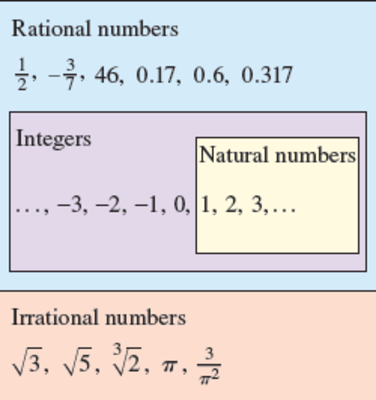## Precalculus: Mathematics for Calculus, 7th Edition

a.) 100 b.) 0, 100, -8 c.) -1.5, 0, $\frac{5}{2}$, 2.71, 3.1$\overline{4}$, 100, -8 d.) $\sqrt 7$, -$\pi$
Natural numbers are integers and integers are rational numbers. The numbers included (-1.5, 0, $\frac{5}{2}$, 2.71, 3.1$\overline{4}$, 100, -8, $\sqrt 7$, -$\pi$ ) can be categorized in the sections a.) Natural numbers - whole Positive integers greater than zero (Ex. 1, 2, 3, 4...). This only includes 100. b.) Integers - every whole number (ex. -1, 0, 1, 2...) This includes 0, 100, and -8 c.) Rational Numbers- any number that is whole or fractional (Ex. $\frac{2}{5}$ , 7). This includes -1.5, 0, $\frac{5}{2}$, 2.71, 3.1$\overline{4}$, 100, and -8 d.)Irrational Numbers- Numbers that cannot be expressed as a ratio of integers (Ex. $\sqrt 2$, $\pi$). This is separate for the rational number section. This only includes $\sqrt 7$ and -$\pi$